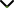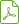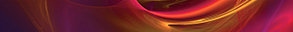# Fuzzy Calculus Theory and Its Applications

Publishing date
17 Nov 2017
Status
Published
30 Jun 2017

Guest Editors

1Al Balqa’ Applied University, Al-Salt, Jordan

2Polytechnic Institute of Porto, Porto, Portugal

3University of Santiago de Compostela, Santiago de Compostela, Spain

4Ondokuz Mayıs University, Samsun, Turkey

# Fuzzy Calculus Theory and Its Applications

## DescriptionFuzzy calculus is the study of theory and applications of integrals and derivatives of uncertain functions. This branch of mathematical analysis, extensively investigated in recent years, has emerged as an effective and powerful tool for the mathematical modeling of several engineering and scientific phenomena.

Based on the wide applications in engineering and sciences such as physics, mechanics, chemistry, and biology, research on fuzzy ordinary and partial differential equations and other relative topics is active and extensive around the world. In the past few years, the increase of the subject is witnessed by hundreds of research papers, several monographs, and many international conferences.

This special issue will be a devoted topic to high current interest falling within the scope of the journal and will attract many papers of the highest quality. The objective of this special issue is to highlight the importance of fuzzy operators and their applications and let the readers of this journal know about the possibilities of this new tool and to provide a multidisciplinary forum of discussions among scientists who have been interested in investigating diverse branches related to fuzzy calculus and applying theories of fuzzy calculus to their diverse research areas.

This issue is expected to welcome articles of significant and original results and review articles of exceptional merit, which are closely related to fuzzy calculus in either theoretical or applicative sense. Also, research articles that are related to the fuzzy complex intelligent systems such as fuzzy artificial neural networks and neurofuzzy systems are also welcome.

Potential topics include but are not limited to the following:

• Development of analytical or numerical methods applied to the solutions of fuzzy differential, integrodifferential, and integral equations that have applications in physics, medicine, mechanics, and so on
• Fuzzy stochastic differential equations and their applications
• Mathematical analysis of fuzzy epidemic models
• Fuzzy difference equations and their applications
• Fuzzy integral and differential inequalities
• Fuzzy artificial neural networks and neurofuzzy systemsDownload Special IssuePublishing CollaborationJournal metricsAuthor guidelinesEditorial boardDatabases and indexing